Univerza na Primorskem Fakulteta za matematiko, naravoslovje in informacijske tehnologije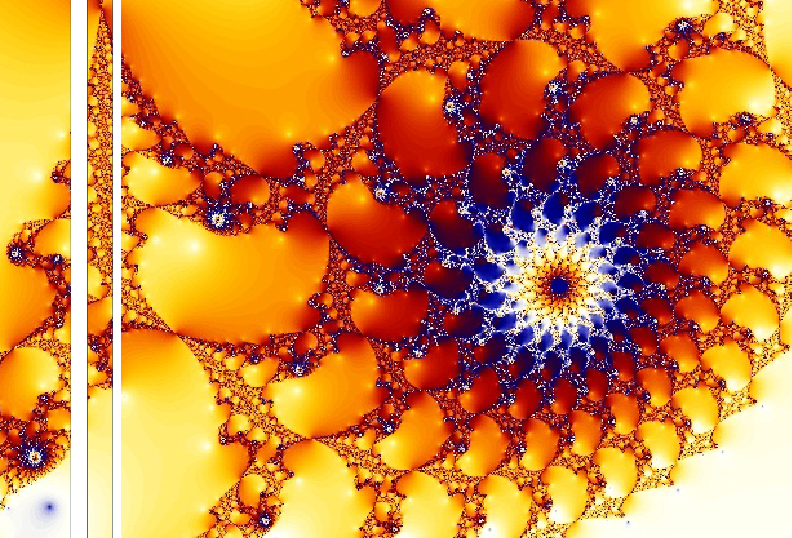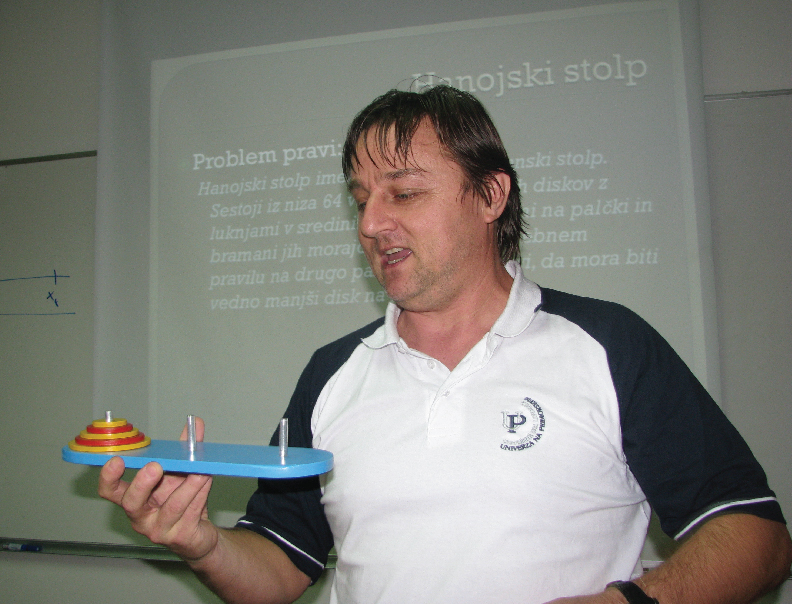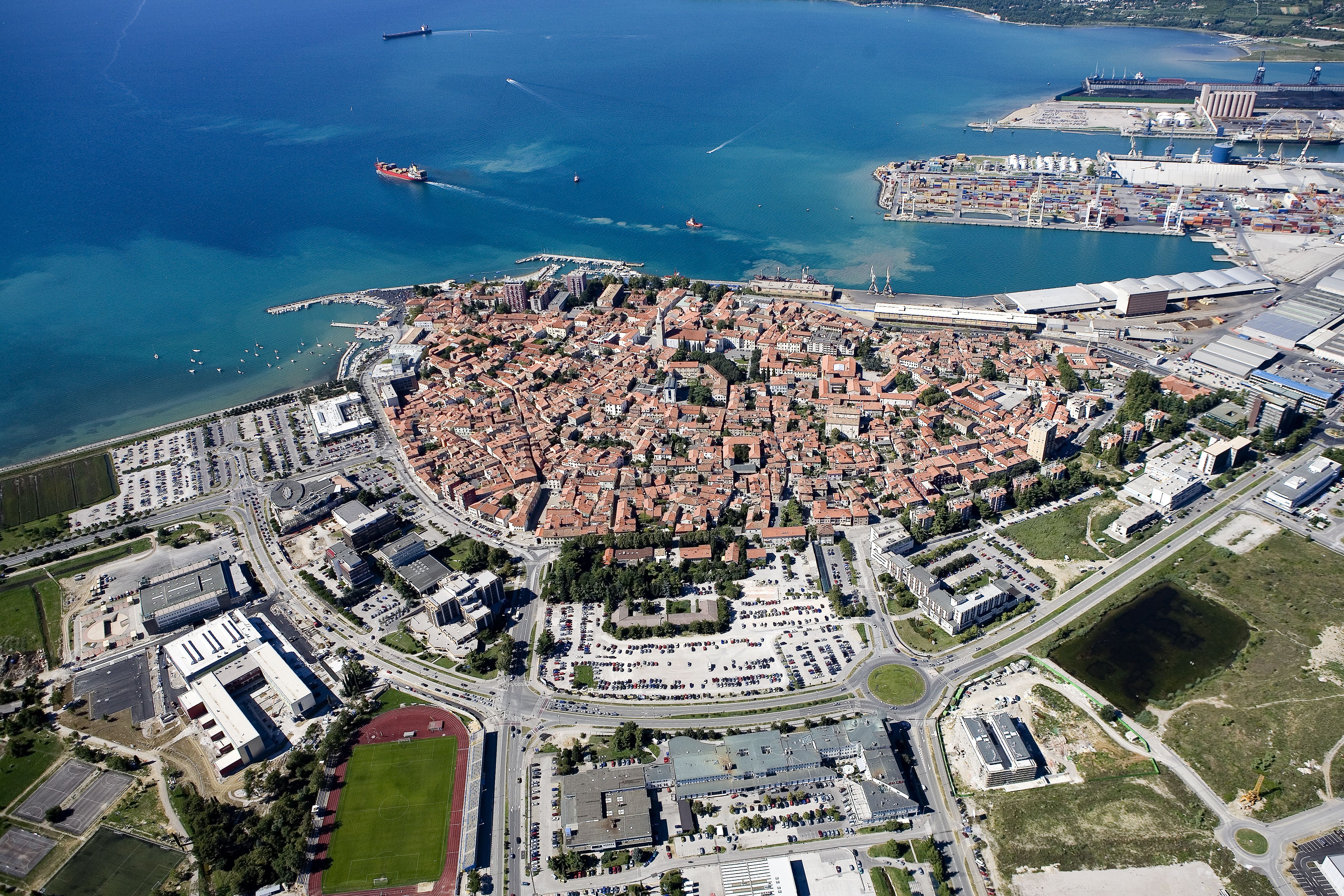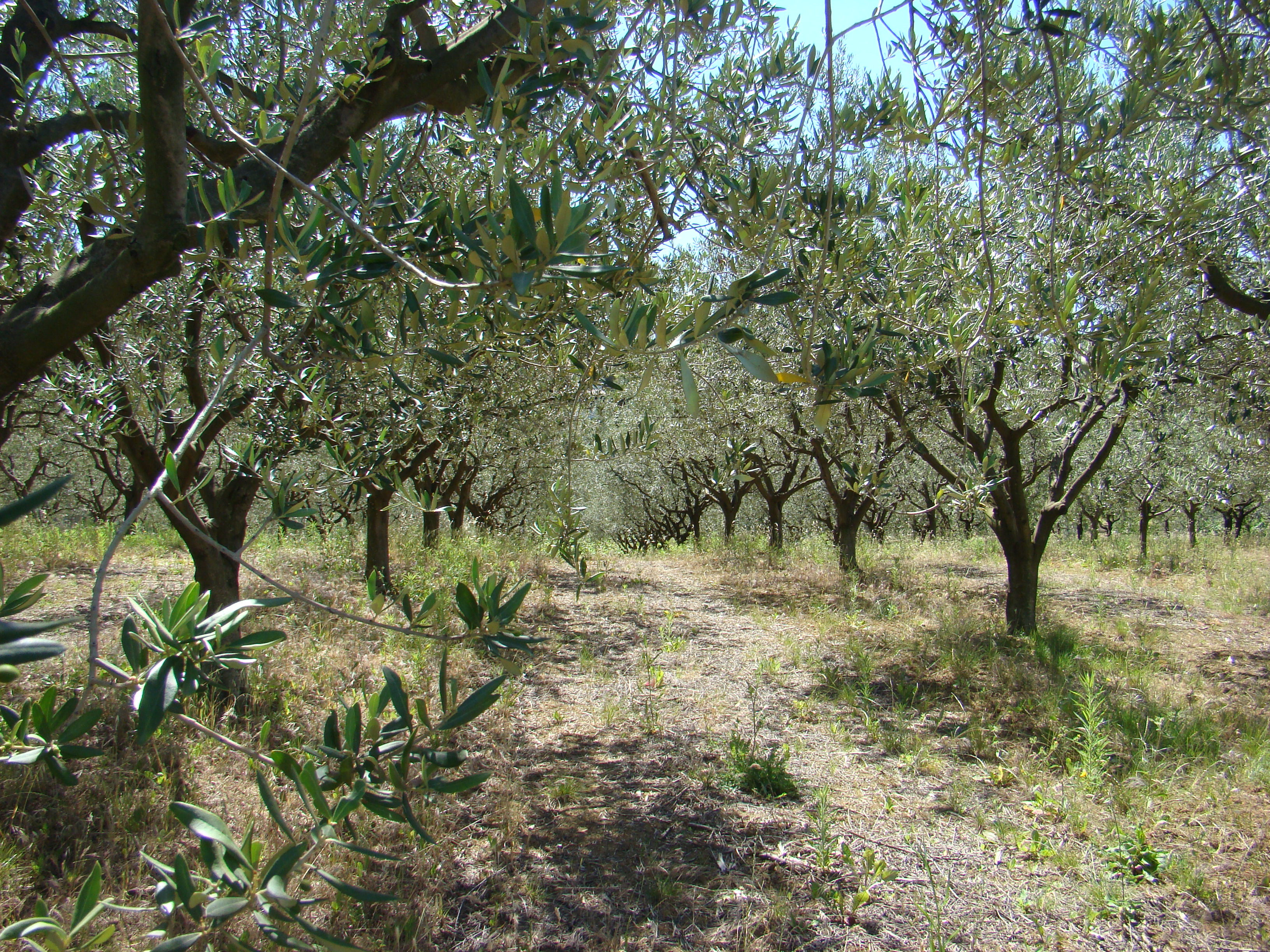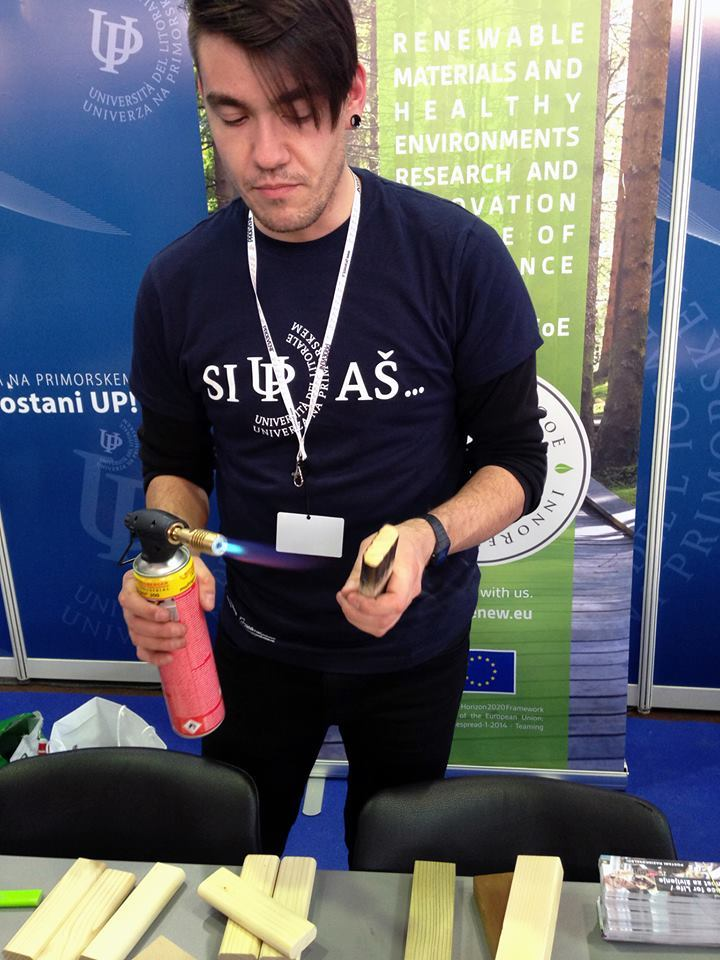SI | EN# Raziskovalni matematični seminar - Arhiv

 2021 2020 2019 2018 2017 2016 2015 2014 2013 2012 2011 2010
 1 2 3 4 5 6 7 8 9 10 11 12
Datum in ura / Date and time: 6.10.14
(11:00-12:00)
Predavalnica / Location: FAMNIT-SEMIN
Predavatelj / Lecturer: Dr. Pablo De Caria (National University of La Plata, Argentina)
Naslov / Title: On chordal and dually chordal graphs and their tree representations
Vsebina / Abstract:

Chordal and dually chordal graphs are well known classes, whose duality becomes evident by the use of trees: a dually chordal graph is characterized by the existence of a tree such that every clique of the graph induces a subtreee (the compatible tree), whereas a chordal graph is characterized by the existence of a tree such that each member of the dual of the clique family induces a subtree (the clique tree).

In this talk, clique trees and compatible trees are analyzed in more depth, thus strengthening the sense of duality between the two classes.

This work is mostly based on some chapters of my PhD thesis, under the supervision of Marisa Gutierrez.

## Download slides.

Datum in ura / Date and time: 6.10.14
(10:00-11:00)
Predavalnica / Location: FAMNIT-SEMIN
Predavatelj / Lecturer: Dr. Flavia Bonomo (Buenos Aires University, Argentina)
Naslov / Title: Minimum weight clique cover in claw-free perfect graphs
Vsebina / Abstract:

For a perfect graph G, and given a weight function w on the vertices of G, linear programming duality ensures that the weight of a minimum weighted clique cover (an assignment of a non-negative weight y_C to each clique C of G, such that, each vertex v is covered by cliques whose weight sums at least w(v) and that
minimizes the total sum of the weights of the cliques) is the same as the weight of a maximum weighted stable set (a set of pairwise nonadjacent vertices S that maximizes the sum of the weights). Moreover Grötschel, Lovász and Schrijver gave in 1988 a (non combinatorial) algorithm, building upon Lovász's theta function, to compute both solutions. It is a major open problem in combinatorial optimization whether there exist polynomial time combinatorial algorithms for those two problems. For particular classes of perfect graphs, such algorithms exist. This is the case, for instance, for claw-free perfect graphs (a graph is claw-free if none of its vertices has a stable set of size three in its neighborhood).

In this talk we will briefly survey the classical algorithms from 1984 due to Hsu and Nemhauser that solve the weighted and the unweighted minimum clique cover problem on claw-free perfect graphs in O(|V(G)|^5) time, and present a very recent approach that reduces the problem to the question whether a given maximal (not necessarily maximum) stable set S, is of maximum weight. In the cardinality case, we answer this question by solving a suitable 2-SAT instance, and obtain a new combinatorial algorithm that produces both a minimum clique cover and an maximum stable set of a claw-free perfect graph G in time O(|V(G)|^3), in the spirit of the augmenting path algorithm for maximum bipartite matching and minimum vertex cover. In the weighted case, the question reduces to a kind of "weighted" 2-SAT problem. This latter problem was first solved by Schrijver in 1991 (and also Peis in 2007), and then independently considered by several people in the Constraint Programming Community, like for instance Schutt and Stuckey in 2010. We propose here a novel approach that takes advantage of the structure of the polyhedron when dealing with the minimum weight clique cover problem. It follows that a minimum weight clique cover of a claw-free perfect graph G can be found in time O(|V(G)|^3).

This is joint work with Gianpaolo Oriolo, Claudia Snels and Gautier Stauffer.

## Download slides.

Datum in ura / Date and time: 29.9.14
(10:00-11:00)
Predavalnica / Location: FAMNIT-SEMIN
Predavatelj / Lecturer: Dr Matthew Johnson (Durham University, UK)
Naslov / Title: Finding (Shortest) Paths between Graph Colourings
Vsebina / Abstract:

The reconfiguration graph of the \$k\$-colourings of a graph \$G\$ contains as its vertex set the proper vertex \$k\$-colourings of \$G\$, and two colourings are joined by an edge in the reconfiguration graph if they differ in colour on just one vertex of \$G\$.  We investigate the structure of reconfiguration graphs and the computational complexity of recognizing whether or not a pair of colourings belong to the same component of the reconfiguration graph (that is, the complexity of deciding if it is possible to transform one colouring into another with a sequence of ''recolouring'' steps that each change the colour on just one vertex without ever breaking the constraint that neighbours are not coloured alike).

We can similarly define the reconfiguration graph (or solution graph) of an instance of any search problem - the vertex set is the set of all possible solutions and the edge relation describes when a pair of distinct solutions have minimum difference (in some sense). We review research into these graphs with a focus on our recent work on shortest paths (in the reconfiguration graph) between graph colourings.
Joint work with Dieter Kratsch, Stefan Kratsch, Viresh Patel and Daniël Paulusma.